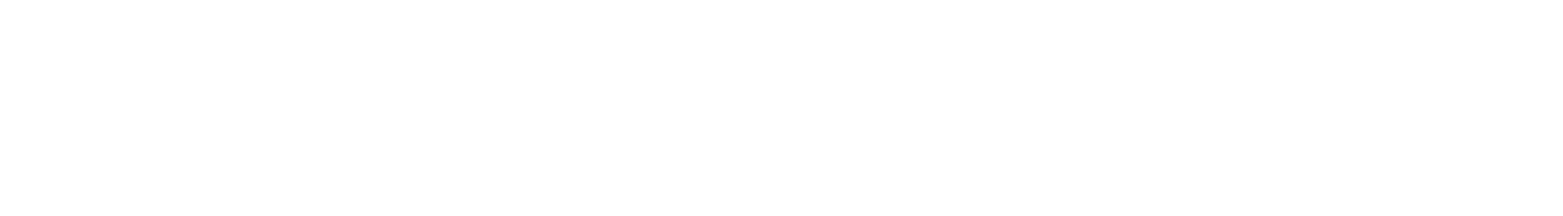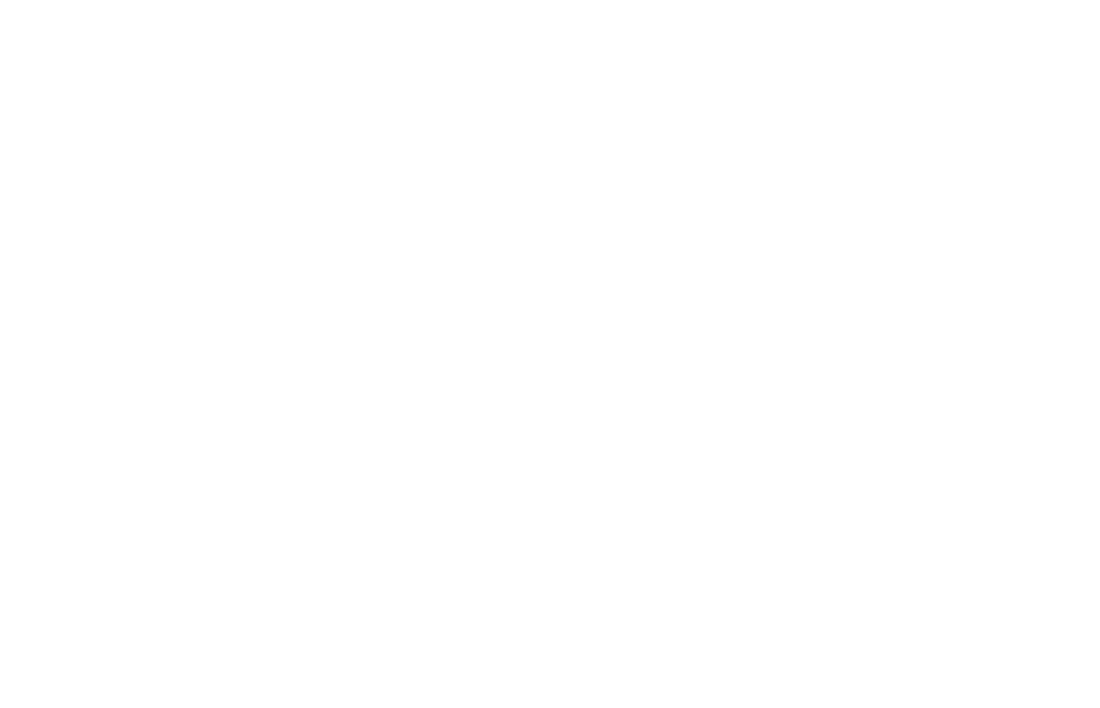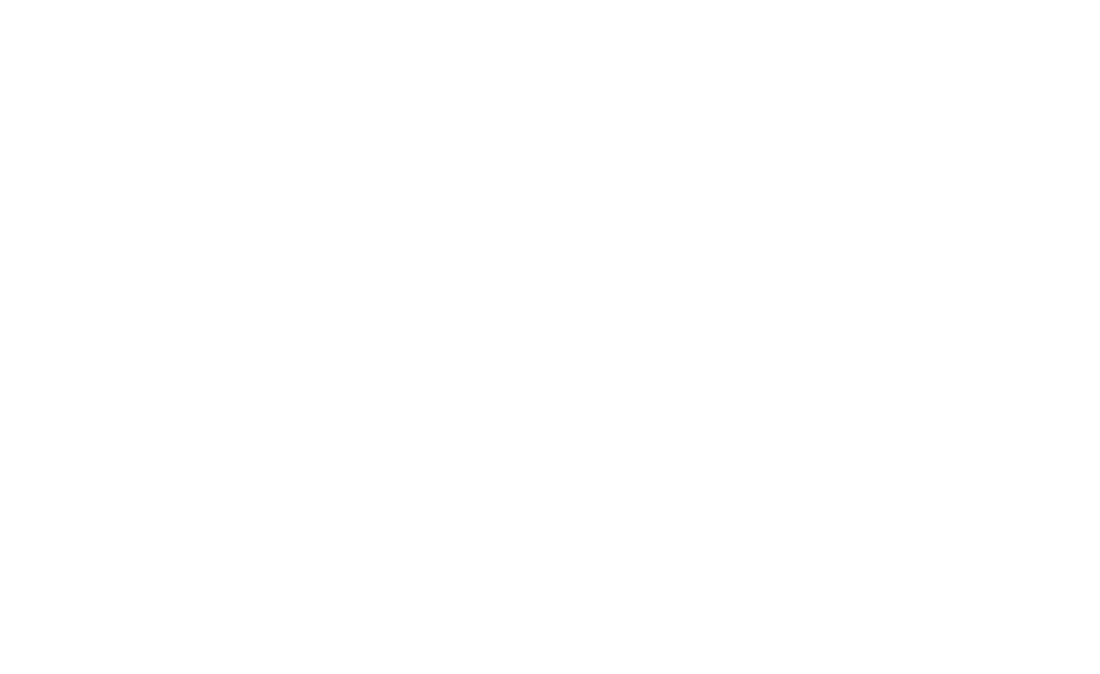1.5.1 Word & Chemical Equations

Writing Equations

Nothing created – nothing destroyed

• New substances are made during chemical reactions
• However, the same atoms are always present before and after reaction
• They have just joined up in different ways
• Atoms cannot be created or destroyed, so if they exist in the reactants then they absolutely must be in the products!
• Because of this the total mass of reactants is always equal to the total mass of products
• This idea is known as the Law of Conservation of Mass

Conservation of Mass

• The Law of Conservation of Mass enables us to balance chemical equations, since no atoms can be lost or created
• You should be able to:
• Write word equations for reactions outlined in these notes
• Write formulae and balanced chemical equations for the reactions in these notes

Word Equations

• These show the reactants and products of a chemical reaction using their full chemical names
• The reactants are those substances on the left-hand side of the arrow and can be thought of as the chemical ingredients of the reaction
• They react with each other and form new substances
• The products are the new substances which are on the right-hand side of the arrow
• The arrow (which is spoken as “goes to” or “produces”) implies the conversion of reactants into products
• Reaction conditions or the name of a catalyst (a substance added to make a reaction go faster) can be written above the arrow
• An example is the reaction of sodium hydroxide (a base) and hydrochloric acid produces sodium chloride (common table salt) and water:

sodium hydroxide + hydrochloric acid ⟶ sodium chloride + water

Representing reactions as equations

• Chemical equations use the chemical symbols of each reactant and product
• When balancing equations, there has to be the same number of atoms of each element on either side of the equation in accordance with the Law of Conservation of Mass
• A symbol equation uses the formulae of the reactants and products to show what happens in a chemical reaction
• A symbol equation must be balanced to give the correct ratio of reactants and products:

S + O2 → SO2

• This equation shows that one atom of sulfur (S) reacts with one  molecule of oxygen (O2) to make one molecule of sulfur dioxide (SO2)
• The following non-metals must be written as molecules: H2, N2, O2, F2, Cl2, Br2 and I2
• To balance an equation you work across the equation from left to right, checking one element after another
• If there is a group of atoms, for example a nitrate group (NO3) that has not changed from one side to the other, then count the whole group as one entity rather than counting the individual atoms
• Examples of chemical equations:
• Acid-base neutralisation reaction:

NaOH (aq) + HCl (aq)  ⟶ NaCl (aq) + H2O (l)

• Redox reaction:

2Fe2O(aq) + 3C (s) ⟶ 4Fe (s) + 3CO2 (g)

• In each equation there are equal numbers of each atom on either side of the reaction arrow so the equations are balanced
• Don’t forget to add state symbols when writing balanced equations:Balancing Equations

The best approach is to practice lot of examples of balancing equations

• By trial and error change the coefficients (multipliers) in front of the formulae, one by one checking the result on the other side
• Balance elements that appear on their own, last in the process

Worked Example

Example 1

Balance the following equation:

aluminium + copper(II)oxide ⟶ aluminium oxide + copper

Unbalanced symbol equation:

Al + CuO ⟶ Al2O3 + CuWorked Example

Example 2

Balance the following equation:

magnesium oxide + nitric acid ⟶ magnesium nitrate + water

Unbalanced symbol equation:

MgO + HNO3 ⟶ Mg(NO3)2 + H2OExam Tip

Chemical equations do not contain an equals sign between the left and right-hand sides but are written with an arrow instead. The arrow means that the reactants have reacted together and formed the product(s).Author: Francesca

Fran has taught A level Chemistry in the UK for over 10 years. As head of science, she used her passion for education to drive improvement for staff and students, supporting them to achieve their full potential. Fran has also co-written science textbooks and worked as an examiner for UK exam boards.
CloseClose